# Generating Random Smooth Curves Using Physics Principles

Here is a general process for making random smooth curves using physics principles:

1) Initialize a particle at the origin with some random velocity.
2) Apply a constant force which is orthogonal to the velocity.
3) Numerically integrate to calculate and plot the trajectory
4) Change the magnitude / sign of the force periodically, while maintaining the orthogonality

This ensures that the particle maintains a constant speed while continuously and smoothly changing its direction.

Plots and source code are below: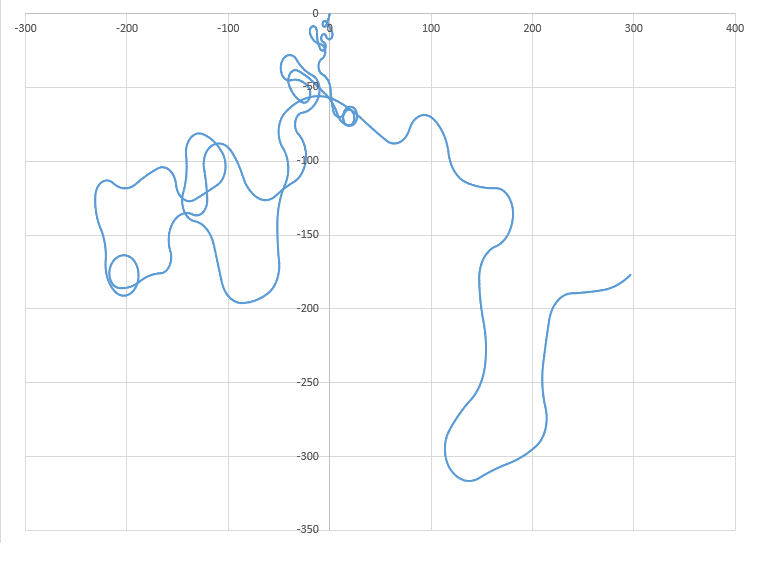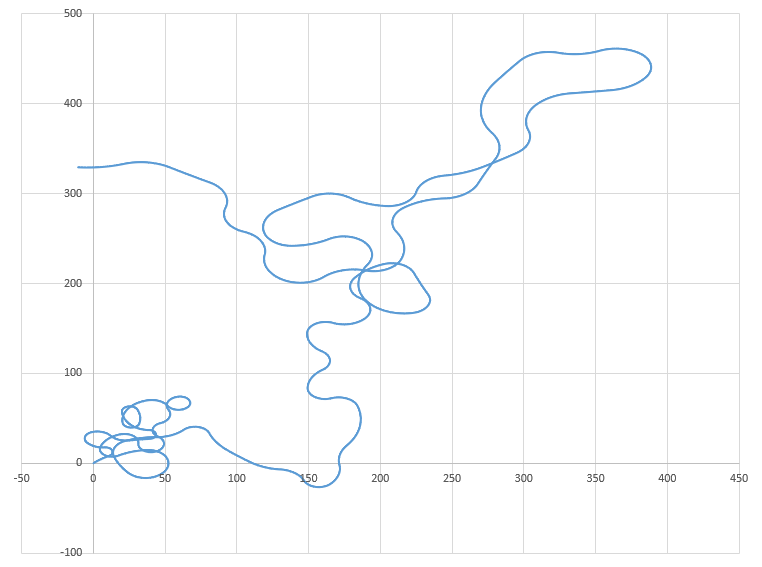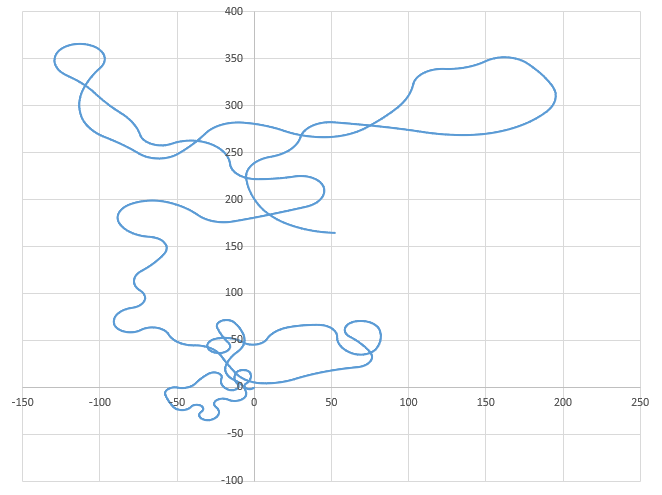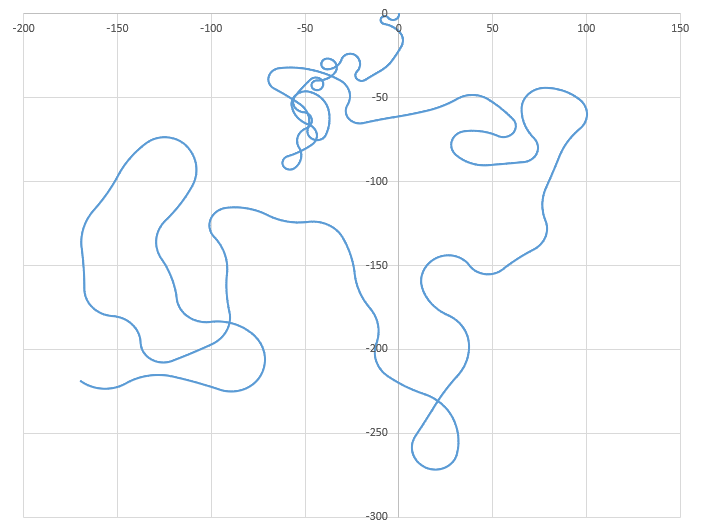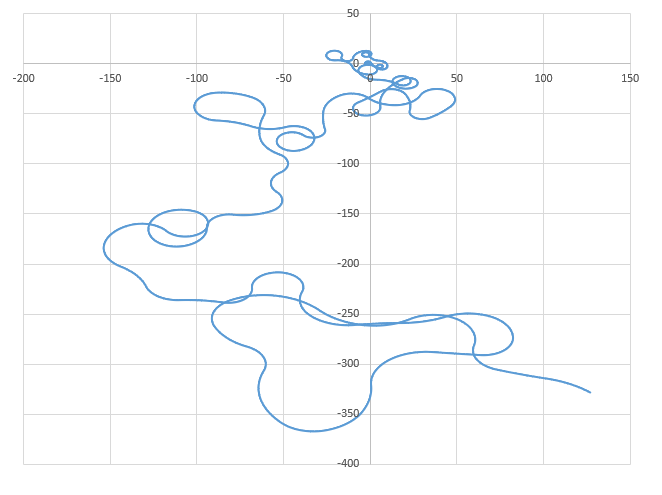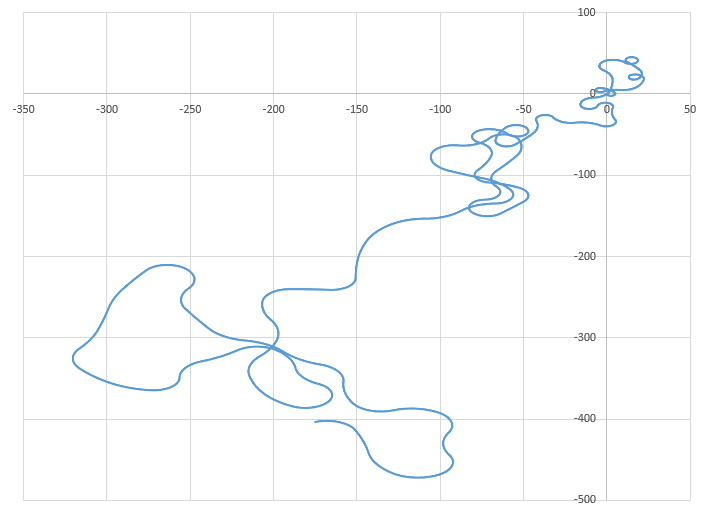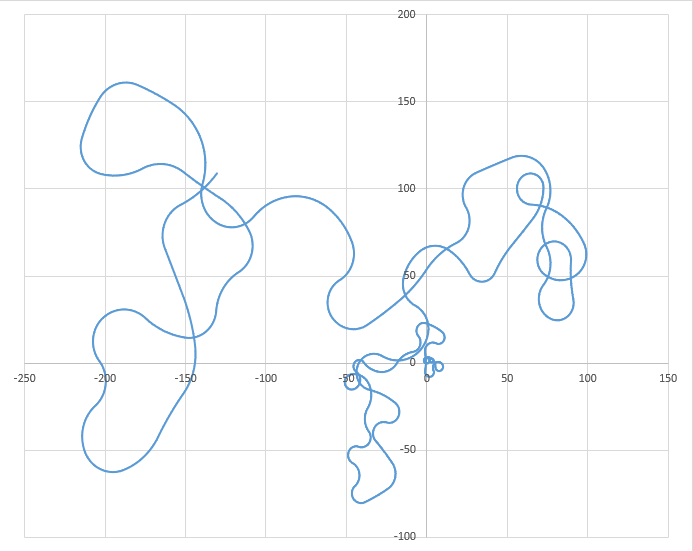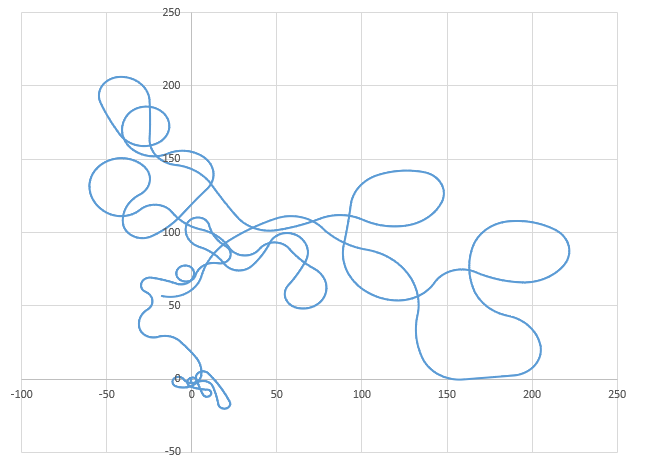import math
import random

x = 0.0
y = 0.0

m = 1.0

vx = -10.0 + 20.0 * random.random()
vy = -10.0 + 20.0 * random.random()

ax = 0.0
ay = 0.0

t = 0.0
dt = 10.0**(-2.0)

count = 0

Fbase = 50.0

Fmag = -Fbase + 2.0 * Fbase * random.random()

while t <= 100.0:

x = x + vx * dt
y = y + vy * dt

vx = vx + ax * dt
vy = vy + ay * dt

if (count % 100) == 0:

Fmag = -Fbase + 2.0 * Fbase * random.random()

Nx = -vy
Ny = vx

Nmag = math.hypot(vx,vy)

Nx = Nx / Nmag
Ny = Ny / Nmag

Fx = Fmag * Nx
Fy = Fmag * Ny

ax = Fx / m
ay = Fy / m

print t,x,y

t = t + dt
count = count + 1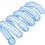Note by Steven Chase
3 years, 6 months ago

This discussion board is a place to discuss our Daily Challenges and the math and science related to those challenges. Explanations are more than just a solution — they should explain the steps and thinking strategies that you used to obtain the solution. Comments should further the discussion of math and science.

When posting on Brilliant:

• Use the emojis to react to an explanation, whether you're congratulating a job well done , or just really confused .
• Ask specific questions about the challenge or the steps in somebody's explanation. Well-posed questions can add a lot to the discussion, but posting "I don't understand!" doesn't help anyone.
• Try to contribute something new to the discussion, whether it is an extension, generalization or other idea related to the challenge.

MarkdownAppears as
*italics* or _italics_ italics
**bold** or __bold__ bold
- bulleted- list
• bulleted
• list
1. numbered2. list
1. numbered
2. list
Note: you must add a full line of space before and after lists for them to show up correctly
paragraph 1paragraph 2

paragraph 1

paragraph 2

[example link](https://brilliant.org)example link
> This is a quote
This is a quote
    # I indented these lines
# 4 spaces, and now they show
# up as a code block.

print "hello world"
# I indented these lines
# 4 spaces, and now they show
# up as a code block.

print "hello world"
MathAppears as
Remember to wrap math in $$ ... $$ or $ ... $ to ensure proper formatting.
2 \times 3 $2 \times 3$
2^{34} $2^{34}$
a_{i-1} $a_{i-1}$
\frac{2}{3} $\frac{2}{3}$
\sqrt{2} $\sqrt{2}$
\sum_{i=1}^3 $\sum_{i=1}^3$
\sin \theta $\sin \theta$
\boxed{123} $\boxed{123}$

Sort by:

This must have taken you forever

- 3 years, 6 months ago

- 3 years, 6 months ago

Wow. You must like coding a lot

- 3 years, 6 months ago

One hour is really reasonable for a programmer.

- 1 year, 3 months ago

what visualization software is that ?

- 3 years, 6 months ago

I'm very primitive. I use Python to print out (x,y) coordinate pairs. And then I paste into Excel and make a scatter plot.

- 3 years, 6 months ago

quite impressive just for that :o

- 3 years, 6 months ago

have you tried what happens with increasing the range ?

- 3 years, 6 months ago

You mean the run time?

- 3 years, 6 months ago

- 3 years, 6 months ago

I probably did, and thought that this was some sort of optimum. I actually wrote the code for this a year ago or so. It might be fun to try a spherical coordinates version too.

- 3 years, 6 months ago

yes exactly, also thought about it. Btw, do you use python 2 or 3 ?

- 3 years, 6 months ago

I use Python 2.7

- 3 years, 6 months ago